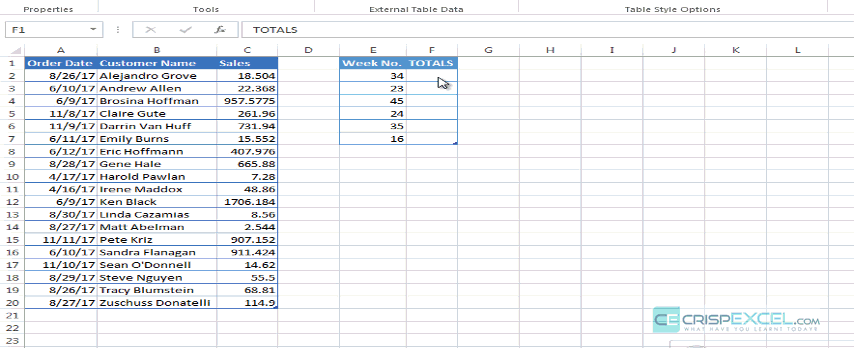# 4 WAYS TO SUM DATA BY WEEK NUMBER

Every organization requires weekly summaries to monitor peak and off-peak periods. This is mostly so when you are in the production section.

Excel has a beautiful but overlooked function (WEEKNUM) that returns an integer representing the week in the year (1 to 53).

For anyone not familiar with WEEKNUM function, it contains 2 arguments; Serial_number ( the date to return the week number for) and an optional Return_type (an integer that defines when the week starts).

NB: If the return type is omitted, function defaults to week start on Sunday all the way to Saturday

`=WEEKNUM( serial_number, [return_type] )`
[return_type] Meaning
1 Week from Sunday to Saturday
2 Week from Monday to Sunday
11 Week  from Monday to Sunday
12 Week from Tuesday to Monday
13 Week from Wednesday to Tuesday
14 Week  from Thursday to Wednesday
15 Week  from Friday to Thursday
16 Week  from Saturday to Friday
17 Week from Sunday to Monday
21 Week  from Monday to Sunday

Beautiful as the function is, it has one major weakness, WEEKNUM  doesn’t accept a range argument e.g. `=WEEKNUM(K11:K110)` just returns #VALUE! error.

So, How do you get week numbers in an array given a range of dates?

Do you need to always use helper column to convert dates to week numbers before any analysis is done?

1. SUMIF
2. SUMPRODUCT
3. SUM & IF
4. PIVOT TABLES

## SUM BY WEEK USING SUMIF

Given below data, Show totals per week.Since WEEKNUM does not accept a range argument, then we have to create a helper column so that we can be able to use SUMIF## SUM BY WEEK USING SUMPRODUCT

It is not entirely true that WEEKNUM does not accept a range argument. It can be forced to accept range by adding zero to a range i.e.

`=WEEKNUM(A1:A25+0)`

The above function does not return an error but an array of week numbers from the given dates range.

Since SUMPRODUCT function comfortably handles arrays, we can use this array of week numbers to create a summary as shown below.`=SUMPRODUCT((WEEKNUM(Table13[Order Date]+0)=[@[Week No.]])*Table13[Sales])`

How it works:

►WEEKNUM(Table13[Order Date]+0) returns an array of week numbers for the given data range

`{34;23;23;45;45;24;24;35;16;16;23;35;35;45;23;45;35;34;35}`

►WEEKNUM(Table13[Order Date]+0)=[@[Week No.]] returns an array of TRUE/FALSE based on the week number criteria.

`{TRUE;FALSE;FALSE;FALSE;FALSE;FALSE;FALSE;FALSE;FALSE;FALSE;FALSE;FALSE;FALSE;FALSE;FALSE;FALSE;FALSE;TRUE;FALSE}`

►(WEEKNUM(Table13[Order Date]+0)=[@[Week No.]])*Table13[Sales]  SUMPRODUCT converts the array of TRUE/FALSE into its numeric equivalent of 1/0  when multiplying  it with the sales figure

`{18.504;0;0;0;0;0;0;0;0;0;0;0;0;0;0;0;0;68.81;0}`
`=SUMPRODUCT({18.504;0;0;0;0;0;0;0;0;0;0;0;0;0;0;0;0;68.81;0})=87.314`

## SUM BY WEEK USING SUM & IF FUNCTION

This is an array function that uses the same principles as SUMPRODUCT

`{=SUM(IF(WEEKNUM(Table135[Order Date]+0)=[@[Week No.]],Table135[Sales]))}`

How it works:

►WEEKNUM(Table13[Order Date]+0)=[@[Week No.]] returns an array of TRUE/FALSE based on the week number criteria.

`{TRUE;FALSE;FALSE;FALSE;FALSE;FALSE;FALSE;FALSE;FALSE;FALSE;FALSE;FALSE;FALSE;FALSE;FALSE;FALSE;FALSE;TRUE;FALSE}`

►IF function returns only the Sales values if the test is TRUE, otherwise returns FALSE

`{18.504;FALSE;FALSE;FALSE;FALSE;FALSE;FALSE;FALSE;FALSE;FALSE;FALSE;FALSE;FALSE;FALSE;FALSE;FALSE;FALSE;68.81;FALSE}`

►Since SUM function ignores texts, It just sums up the numbers

`=SUM({18.504;FALSE;FALSE;FALSE;FALSE;FALSE;FALSE;FALSE;FALSE;FALSE;FALSE;FALSE;FALSE;FALSE;FALSE;FALSE;FALSE;68.81;FALSE})=87.314`

## SUM BY WEEK USING PIVOT TABLES

This is the simplest and easiest method.

Its only major drawback is that it will give you the weeks date range but not the week number.

NB: The trick in using pivot table is knowing how to group the dates into weeks. Watch the video again.

## Conclusion

There is now no need to lose your hair over worrying how to do weekly summaries.

You can use the same techniques taught above to do Average and Count.

If I have missed something, share.